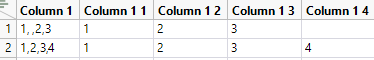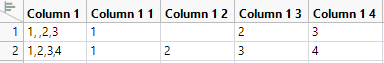Our World Statistics Day conversations have been a great reminder of how much statistics can inform our lives. Do you have an example of how statistics has made a difference in your life? Share your story with the Community!
Choose Language Hide Translation Bar
Highlighted

## Cols utilities: how to let <text to columns> take care of empty data?

Hi

I got a problem when using Cols->utilities->text to columns.

For example I have data 1, ,2,3, after doing text to columns, I want to get {1},{ },{2},{3} these four columns, while JMP gives me {1},{2},{3} as below screen shot.

Excel can do this with <Text to Columns>

Is there an solution out there in JMP?

The result I got from JMP:what I expected:1 ACCEPTED SOLUTION

Accepted Solutions
Highlighted

## Re: Cols utilities: how to let <text to columns> take care of empty data?

I am not aware of doing what you want with the Text to Columns() function, but here is a simple function that will do what you want

``````Names Default To Here( 1 );
dt = New Table( "Example",
New Column( "string",
Character,
"Nominal",
Set Values( {"1,2,3", "1,2,3,4", "1,,3,4"} ),
Set Display Width( 44 )
)
);

newTexttoColumns = Function({dt,x},{defaultlocal},
start = N Cols( dt );
For( theRow = 1, theRow <= N Rows( dt ), theRow++,
theWord = "";
wordCnt = 1;
For( i = 1, i <= Length( Column( x )[theRow] ), i++,

If( Substr( Column( x )[theRow], i, 1 ) == "," | i == Length( Column( x )[theRow] ),
If(i == Length( Column( x )[theRow]),theWord = theWord || Substr( Column( x )[theRow], i, 1 ));
If( N Col( dt ) < start + wordCnt,
dt << New Column( "", character )
);
Column( start + wordCnt )[theRow] = theWord;
wordCnt++;
theWord = "";
,
theWord = theWord || Substr( Column( x )[theRow], i, 1 )
)

);
);
0;
);

return = newTexttoColumns(dt,"string");``````
Jim
3 REPLIES 3
Highlighted

## Re: Cols utilities: how to let <text to columns> take care of empty data?

I am not aware of doing what you want with the Text to Columns() function, but here is a simple function that will do what you want

``````Names Default To Here( 1 );
dt = New Table( "Example",
New Column( "string",
Character,
"Nominal",
Set Values( {"1,2,3", "1,2,3,4", "1,,3,4"} ),
Set Display Width( 44 )
)
);

newTexttoColumns = Function({dt,x},{defaultlocal},
start = N Cols( dt );
For( theRow = 1, theRow <= N Rows( dt ), theRow++,
theWord = "";
wordCnt = 1;
For( i = 1, i <= Length( Column( x )[theRow] ), i++,

If( Substr( Column( x )[theRow], i, 1 ) == "," | i == Length( Column( x )[theRow] ),
If(i == Length( Column( x )[theRow]),theWord = theWord || Substr( Column( x )[theRow], i, 1 ));
If( N Col( dt ) < start + wordCnt,
dt << New Column( "", character )
);
Column( start + wordCnt )[theRow] = theWord;
wordCnt++;
theWord = "";
,
theWord = theWord || Substr( Column( x )[theRow], i, 1 )
)

);
);
0;
);

return = newTexttoColumns(dt,"string");``````
Jim
Highlighted

## Re: Cols utilities: how to let <text to columns> take care of empty data?

Hi Jim,

What a speed to deliver such a cool script!

Thanks a lot.

BTW, I think JMP may need to consider to enhance the function of <text to columns>.

Highlighted

## Re: Cols utilities: how to let <text to columns> take care of empty data?

If you think JMP should have the enhancement, please add it to the JMP Wish List

Jim
Article Labels

There are no labels assigned to this post.